# Class 9 RD Sharma Solutions – Chapter 10 Congruent Triangles- Exercise 10.3

### Question 1. In two right triangles, one side an acute angle of one are equal to the corresponding side and angle of the other. Prove that the triangles are congruent.

Solution:

We have two right triangles ABC and DEF in which one side and acute angle of one are equal to the corresponding side and angles of the other, i.e.,

∠A = ∠D

BC = EF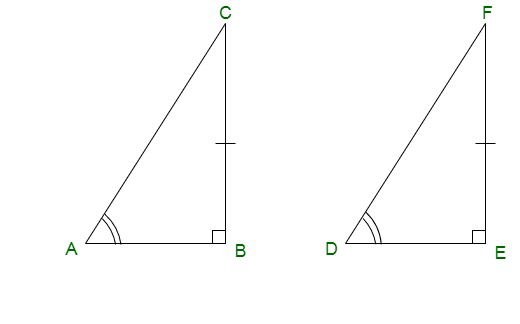Prove: Δ ABC ≅ Δ DEF

Proof:

From Δ ABC and Δ DEF

∠ B = ∠ E = 90° …….(i)[Right triangle]

BC = EF …….(ii)[Given]

∠A = ∠D ……(iii)[Given]

By AAS congruence criterion,

Δ ABC ≅ Δ DEF

Hence, proved.

### Question 2. If the bisector of the exterior vertical angle of a triangle be parallel to the base. Show that the triangle is isosceles.

Solution:

In ABC be a triangle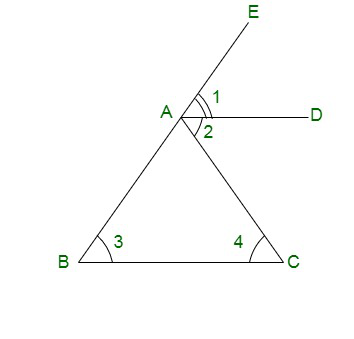From the above figure,

∠1 = ∠2 [AD is a bisector of ∠ EAC]

∠1 = ∠3 [Corresponding angles]

and

∠2 = ∠4 [alternative angle]

Also, we have ∠3 = ∠4

AB = AC

So the sides AB and AC are equal so

Δ ABC is an isosceles triangle.

### Question 3. In an isosceles triangle, if the vertex angle is twice the sum of the base angles, calculate the angles of the triangle.

Solution:

Let us considered Δ ABC is isosceles triangle

In which AB = AC and ∠B = ∠C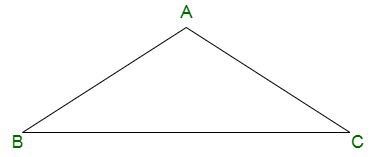Given: ∠A = 2(∠B + ∠C)

Find: The value of ∠A, ∠B, and ∠C

So, we have

∠A = 2(∠B + ∠C)

∠A = 2(∠B + ∠B) [∠B = ∠C because Δ ABC is isosceles triangle]

∠A = 2(2 ∠B)

∠A = 4(∠B)

As we know that sum of angles in an isosceles triangle = 180°

So, ∠A + ∠B + ∠C = 180°

Now put the value of ∠A and ∠C, we get

4 ∠B + ∠B + ∠B = 180°

6 ∠B =180°

∠B = 30°

Here, ∠B = ∠C

∠B = ∠C = 30°

And ∠A = 4 ∠B

∠A = 4 x 30° = 120°

Hence, the value of ∠A = 120°, ∠B = 30°, and ∠C = 30°.

### Question 4. PQR is a triangle in which PQ = PR and is any point on the side PQ. Through S, a line is drawn parallel to QR and intersecting PR at T. Prove that PS = PT.

Solution:

In ΔPQR is a triangle

It is given that PQ = PR, and S, a line is drawn parallel to QR and intersecting PR at T

So, ST || QR.

Prove: PS = PT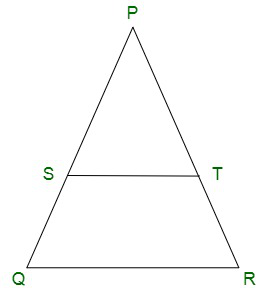As we know that PQ = PR, so the given △PQR is an isosceles triangle.

Hence, ∠ PQR = ∠ PRQ

∠ PST = ∠ PQR and ∠ PTS = ∠ PRQ [Corresponding angles as ST parallel to QR]

∠ PQR = ∠ PRQ

∠ PST = ∠ PTS

So, In Δ PST,

∠ PST = ∠ PTS

Therefore, Δ PST is an isosceles triangle.

So, PS = PT.

Hence, proved.

### Question 5. In an △ABC, it is given that AB = AC and the bisectors of B and C intersect at O. If M is a point on BO produced, prove that ∠MOC = ∠ABC.

Solution:

In △ABC,

It is given that AB = AC and the bisector of ∠B and ∠C intersect at O.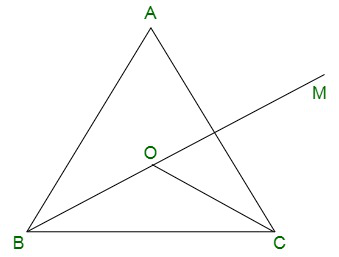Prove: ∠MOC = ∠ABC

It is given that AB = AC

So the △ABC is an isosceles triangle

Hence

∠B = ∠C

∠ABC = ∠ACB

From the figure BO and CO are bisectors of ∠ABC and ∠ACB

So,

∠ABO = ∠OBC = ∠ACO = ∠OCB = 1/2 ∠ABC = 1/2 ∠ACB ………..(i)

In △OBC

∠BOC + ∠MOC = 180° ………(ii) [Straight line]

∠OBC + ∠OCB + ∠BOC = 180°[Sum of angles in an isosceles triangle = 180°]

From equation (ii)

∠OBC + ∠OCB + ∠BOC = ∠BOC + ∠MOC

∠OBC + ∠OCB = ∠MOC

2∠OBC = ∠MOC

From Equation(i)

2(1/2 ∠ABC) = ∠MOC

∠ABC = ∠MOC

Hence proved

### Question 6. P is a point on the bisector of an angle ABC. If the line through P parallel to AB meets BC at Q, prove that triangle BPQ is isosceles.

Solution:

Given that P is a point on the bisector of ∠ABC, and PQ || AB.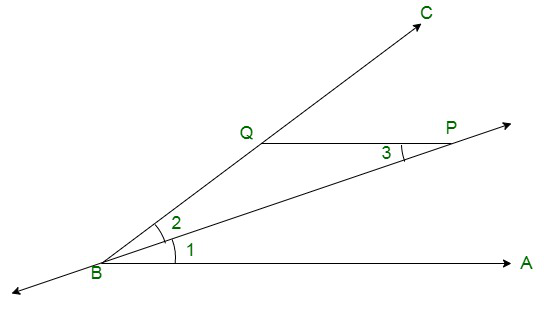Prove: △BPQ is an isosceles triangle

It is given that BP is bisector of ∠ABC

So, ∠ABP = ∠PBC ……….(i)

Also given that PQ || AB

So, ∠BPQ = ∠ABP ……….(ii) [alternative angles]

From equation (i) and (ii), we have

∠BPQ = ∠PBC

or ∠BPQ = ∠PBQ

In △BPQ

∠BPQ = ∠PBQ

Hence, proved △BPQ is an isosceles triangle.

### Question 7. Prove that each angle of an equilateral triangle is 60°.

Solution:

Prove: Each angle of an equilateral triangle is 60°.

Let us considered we have an equilateral triangle △ABC

So, AB = BC = CA

Take AB = BC

So, ∠A = ∠C …….(i) [Because opposite angles to equal sides are equal]

Take BC = AC

∠B = ∠A ………(ii) [Because opposite angles to equal sides are equal]

From (i) and (ii), we get

∠A = ∠B = ∠C ………….(iii)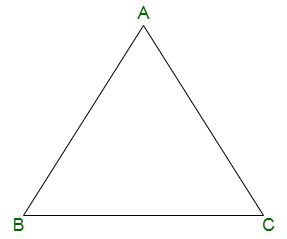As we already know that the sum of angles in a triangle = 180°

So, ∠A + ∠B + ∠C = 180°

From equation(iii), we get

∠A + ∠A + ∠A = 180°

3∠A = 180°

∠A = 60°

So, ∠A = ∠B = ∠C = 60°

Hence Proved

### Question 8. Angles ∠A, ∠B, ∠C of a triangle ABC are equal to each other. Prove that ABC is equilateral.

Solution:

Given that in △ABC

∠A =∠B = ∠C

Prove: △ABC is equilateralIn △ABC

As we already know that the sum of angles in a triangle = 180°

So, ∠A + ∠B + ∠C = 180°

Given that ∠A =∠B = ∠C

So,

∠A + ∠A + ∠A = 180°

3∠A = 180°

∠A = 60°

So, ∠A =∠B = ∠C = 60°

As we know that the angles of equilateral triangles are of 60°

Hence, proved that △ABC is equilateral.

### Question 9. ABC is a triangle in which ∠B = 2 ∠C. D is a point on BC such that AD bisects ∠BAC and AB = CD. Prove that ∠BAC = 72°.

Solution:

Given that in △ABC,

∠B = 2 ∠C, AD bisectors of∠BAC, and AB = CD.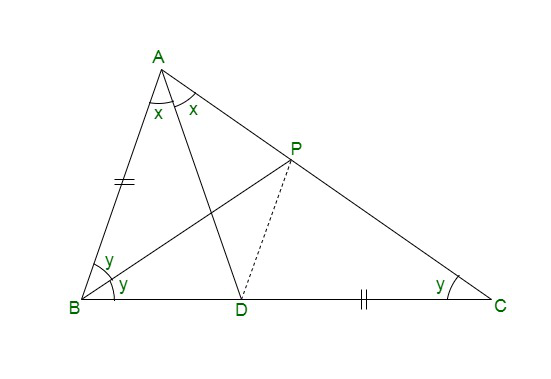Prove:∠BAC = 72°

Now, draw a line BP which is bisector of ∠ABC, and join PD.

Let us considered∠C = ∠ACB = y

∠B = ∠ABC = 2y

Let us considered ∠BAD = ∠DAC = x

∠BAC = 2x [AD is the bisector of ∠BAC]

In △BPC,

∠CBP = ∠PCB = y [BP is the bisector of ∠ABC]

PC = BP

In △ABP and △DCP,

∠ABP = ∠DCP = y

AB = DC [Given]

And PC = BP

So, by SAS congruence criterion,

△ABP ≅ △DCP

∠BAP = ∠CDP and AP = DP [C.P.C.T]

∠CDP = 2x

In △ABD

So,

2y + x = ∠ADP + ∠PDC

2y + x = x + 2x

2y = 2x

y = x

In △ABC,

As we already know that the sum of angles in a triangle = 180°

∠A + ∠B + ∠C = 180°

2x + 2y + y = 180° [∠A = 2x, ∠B = 2y, ∠C = y]

2(y) + 3y = 180° [x = y]

5y = 180°

y = 36°

∠A = ∠BAC = 2 × 36 = 72°

∠A = 72°

Hence proved

### Question 10. ABC is a right-angled triangle in which ∠A = 90° and AB = AC. Find ∠B and ∠C.

Solution:

Given that in ABC is a right-angled triangle

∠A = 90° and AB = AC

Find: ∠B and ∠C

In △ABC

AB = AC [Given]

∠B = ∠C

As we already know that the sum of angles in a triangle = 180°

So, ∠A + ∠B + ∠C = 180°

90° + ∠B + ∠B = 180°

2 ∠B = 180°  – 90°

∠B = 45°

Hence, the value of ∠B = ∠C = 45°.

Whether you're preparing for your first job interview or aiming to upskill in this ever-evolving tech landscape, GeeksforGeeks Courses are your key to success. We provide top-quality content at affordable prices, all geared towards accelerating your growth in a time-bound manner. Join the millions we've already empowered, and we're here to do the same for you. Don't miss out - check it out now!

Previous
Next